×

Search anything:

# Downsampling and Upsampling in CNN

#### Deep Learning cnnFREE BOOK -> Problems for the day before your Coding Interview (on Amazon)

There is no doubt that convolution neural network gave a huge progress to computer vision sector and in this article I will walk with you in short journey with some of its concepts specially downsampling and upsampling in CNN.

### 5. Different ways for upsampling.

In sake of visual understanding of todays concepts i will use the coming fully convolution network.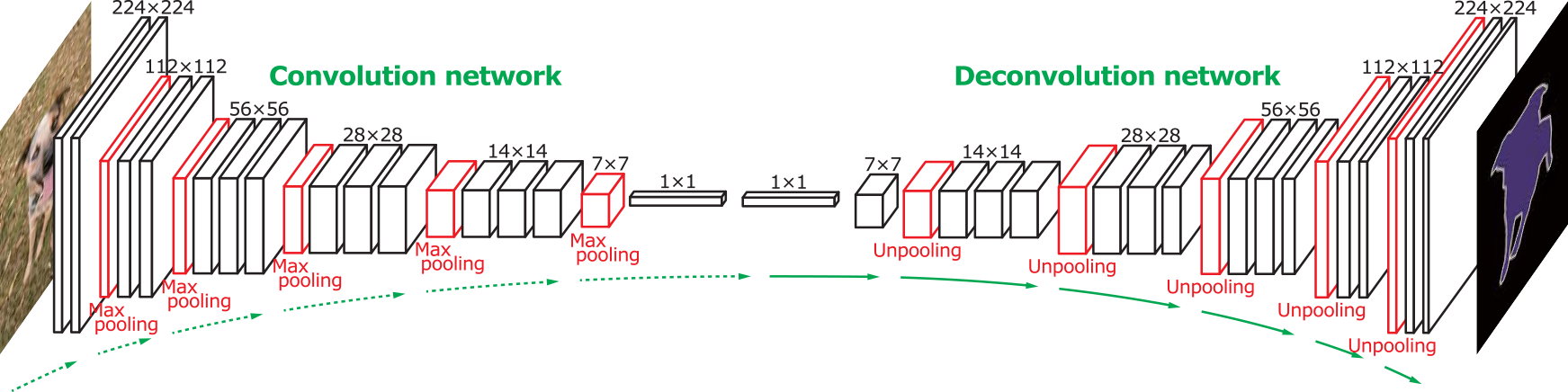### 1. Recap of what CNN is :

Convolution neural network is a technique that trying to extract features from images using filters and then mapping these features maps to a class or a label, Instead of naive DNN or deep neural network that just mapping the simple pixels with the class after a deep network of dense layers. And CNN technique shows a great progress in compare with naive DNN. Here how it work.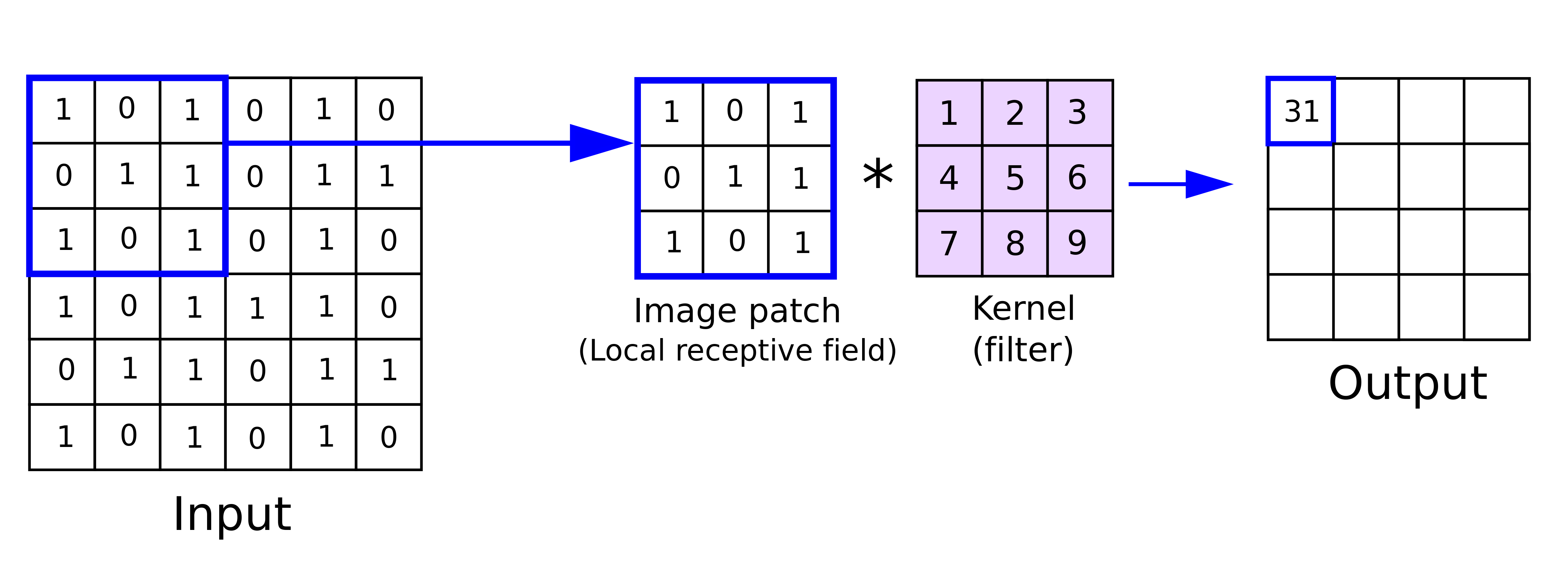A filter of size (f,f) will go on its size of image then element wise multiplication then summation and put the sum as the first pixel of output and moving to the next image patch by moving step called stride (S), some times we use what is calling padding (p) to preserve the output shape as input shape. in this brilliant way we can extract features like vertical or horizontal lines or even circles from an image like this.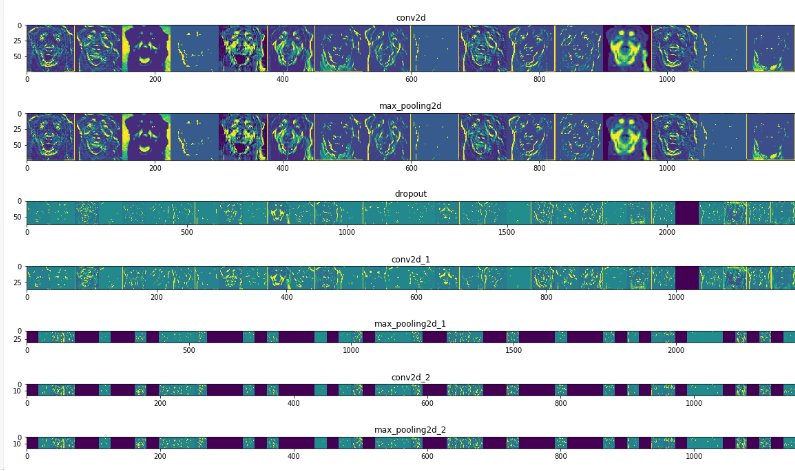### 2. Downsampling in CNN

But what after extracting features from first layer ?! should we enter this output directly to the second convolution layer ?! In fact this will be computationally expensive, So we will prefer to reduce the size of output with minimal effect on features extracted and this what is called Downsampling. And this operation is the first half of most fully convolution networking.

the dimensions of the output will follow this equation :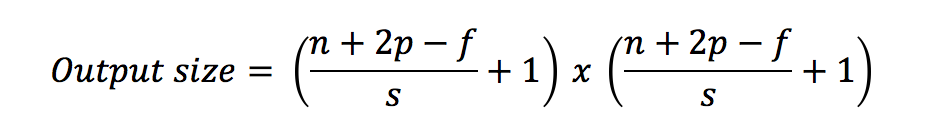and the full shape of the output will follow this :

output shape =batch_shape + (new_rows, new_cols, no.of filters).

where :
n = input height or width
s = stride
f = filter size

So, it is obvious that increasing the denominator (the stride) will lead to shrinkage the output size.

### 3. Different ways for downsampling :

In addition to doing convolution with striding > 1 to reduce output size for the sake of downsampling there is another very famous way for doing this called Polling (max polling is used in most cases than average pooling), In this way we define a pooling size that taking the maximum value over an input window (of size defined by pool_size) (or take the average) also with moving steps (stride). So maxpooling with poo_size of (2,2) and stride of 2 will give output 25% of the input size.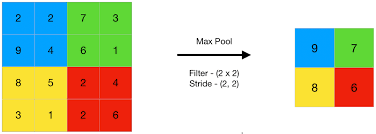These methods are very computationally effective by reducing the size without highly effect of features.(very clear in the image of dogs features).

Simple implementation in keras for the 2 types :

``````
tf.keras.layers.Conv2D(
filters,
kernel_size,
strides=(1, 1),
data_format=None,
dilation_rate=(1, 1),
groups=1,
activation=None,
use_bias=True,
kernel_initializer="glorot_uniform",
bias_initializer="zeros",
kernel_regularizer=None,
bias_regularizer=None,
activity_regularizer=None,
kernel_constraint=None,
bias_constraint=None)
``````

and over write stride > 1 for downsampling.

``````
tf.keras.layers.MaxPooling2D(
``````

### 4. Upsampling in CNN

But what if we want the out put to be in the same size of the input "imagine you are in a semantic segmentation task where every pixel of the input will assigned to a label so you need an output of the same size of input". Meaning we need to reverse the "Downsampling".

Starting with small size image, we need to reach to the previous size. "actually we just need to reach to the same size not to the exact features map", this what is called Upsampling

And this is the second half of fully convolution network that seeking to return to the original size.

There are many way to do this.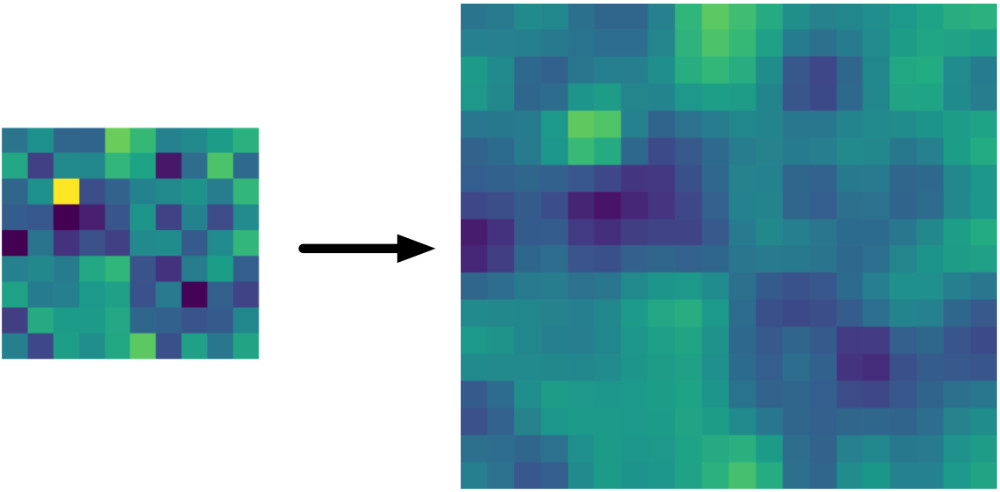### 5. Different ways for upsampling :

• Simple upsampling (Nearest neighbor) :

from its name it is a very simple and computationally cheap operation it just copy or repeat the rows then the columns according to the upsampling factor.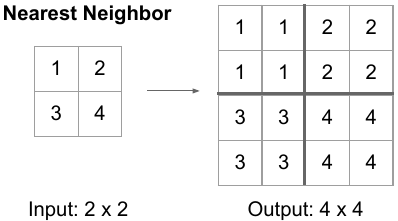Here the upsampling factor is (2,2) so doubling the rows then doubling the columns leading to increase the output size.

With easy implementation in keras :

``````
tf.keras.layers.UpSampling2D(
size=(2, 2), data_format=None, interpolation="nearest", **kwargs
)
``````

where size is the upsampling factor and data_format is where the channel first or last.

• Un-pooling :

Instead of naive repeating of pixels we can just reverse operation used in downsampling, reversing pooling with Un-pooling as following :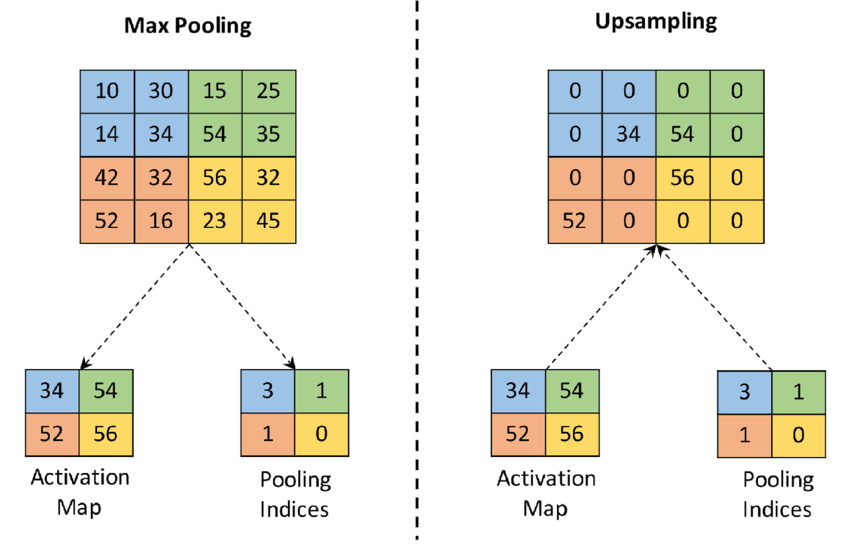Not returning to the same exact pixels but at least to the same resolution with most important pixels.

• Transpose convolution :

OR we can reverse the convolution layers using what is said Transpose convolution or deconvolution "which is mathematically incorrect term" or unconv or partially stride convolution and it is the most common way for upsampling, we just transpose what happened in the regular convolution as following.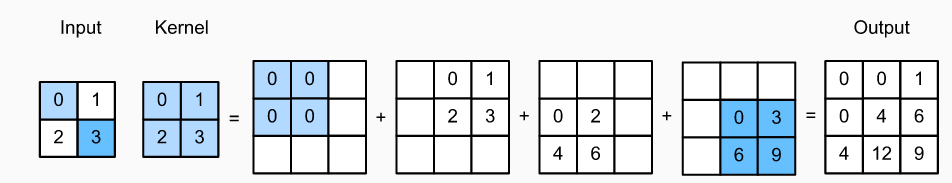each pixel of the input is multiplying with the kernel and put the output(the same as the kernel size) in the final output feature map and moving again with a stride (but this time stride to the output), So here increasing the stride will increase the output size, on the other hand increasing the padding will decrease the output size. Here the output size equation :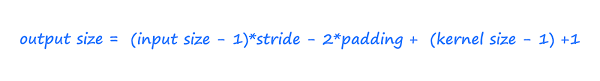As we see the stride and padding have an opposite effect on output size rather than the effect they have in the normal convolution.
And here how you can use in keras:

``````tf.keras.layers.Conv2DTranspose(
filters,
kernel_size,
strides=(1, 1),
data_format=None,
dilation_rate=(1, 1),
activation=None,
use_bias=True,
kernel_initializer='glorot_uniform',
bias_initializer='zeros',
kernel_regularizer=None,
bias_regularizer=None,
activity_regularizer=None,
kernel_constraint=None,
bias_constraint=None)
``````

SUMMARY

Operation Effect on output Types
Downsampling decrease the size standard convolution with stride >1, Pooling (max or average)
Upsampling increase the size nearest neighbor, un-pooling and transpose convolution